### 5.1 The Fundamentals Behind StdRegions

The idea behind standard regions can be traced back to one of the fundamental principles we learn in calculus: differentiation and integrating on arbitrary regions are often difficult; however, differentiation and integration have been worked out on canonical (straight-sided or planar-sided), right-angled, coordinate aligned domains. In the case of Nektar++, we do not need to work on truly arbitrary domains, but rather on seven fundamental domains: segments in 1D, triangles and quadrilaterals in 2D, and tetrahedra, hexahedra, prisms and pyramids in 3D. Since Nektar++ deals with polynomial methods, the natural domain over which reference elements should be build is [-1,1] as this is the interval over which Jacobi Polynomials are defined and over which Gaussian quadrature is defined . We show in Figure 5.1 a pictorial representation of the standard segment, standard quadrilateral (often shorthanded quad) and standard triangle.Figure 5.1 Example of the 1D and 2D reference space elements (segment, quad and triangle).

The standard quad and standard hexahedra (shorthanded ’hex’) are geometrically tensor-product constructions defined on [-1,1]d for d = 2 and d = 3 respectively. The standard triangle is constructed by taking ξ1 ∈ [-1,1] and taking ξ2 ≤-ξ1. The standard tetrahedra (shorthanded ’tet’) is built upon the standard triangle and has all four faces being triangles, with the two triangles along the coordinate directions looking like the standard triangle. The standard prism consists of a standard triangle along the ξ1 --ξ2 plane extruded into the third direction (yielding three quadrilateral faces). The standard pyramid consists of a standard quadrilateral at the base with four triangular faces reaching up to its top vertex We show this pictorially in Figure 5.2.Figure 5.2 Hexahedron to tetrahedron transformation showing how to go from an element on [-1,1]3 (i.e. a standard hexahedron) to the three other element types which are subsets on [-1,1]3. Image taken from .

Regardless of the particular element type, we use the fact one can build polynomial spaces over these geometric objects. In the case of triangles and tetrahedra, these are polynomial spaces in the mathematical sense, i.e. P(k) spaces. In the case of quadrilaterals and hexahedra, these are bi– and tri-polynomial spaces, i.e. Q(k). In the case of prisms and pyramids, the spaces are more complicated and are a mixture in some sense of these spaces, but they are indeed polynomial in form. This allows us to define differentiation exactly and to approximate integrals exactly up to machine precision.

In what is to follow, we describe (1) how you can build differentiation and integration operators over standard regions by mapping them to a domain over which you can exploit index separability; and then (2) how you can build differentiation and integration operators natively over various standard region shapes. The former allows one to benefit from tensor-product operators, and is at present the primary focus of all Nektar++ operators. The latter as implemented for nodal element types is currently an is-a class definition extended from the (tensor-product-based) standard element definitions.

#### 5.1.1 Reference Element Transformations That Facilitate Separability

Differentiation and integration over the standard elements within Nektar++, in general, always try to map things back to tensor-product (e.g. tensor-contraction indexing). This allows one to transform operators that would normally have iterators that go from i = 0,…,Nd where d is the dimension of the shape to operators constructed with d⋅N operations (i.e., the product of one-dimensional operations).

As an example (to help the reader gain an intuitive understanding), consider the integration of a function f(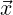) over a hexahedral element. If we had a quadrature rule in 3D that needed Nd points to integrate this function exactly, we would express the operation as: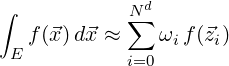where E denotes our d-dimensional element and the set Q = {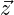ii} denotes the set of points and quadrature weights over the element E. Now, suppose that both our function f(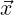) and our Q were separable: that is, f() can be written as f1(x1) ⋅ f2(x2) ⋅ f3(x3) where= (x1,x2,x3)T and Q can we written in terms of 1D quadrature: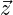i = zi1(1) ⋅ zi2(2) ⋅ zi3(3) with the index handled by an index map σ defined by i = σ(i1,i2,i3) = i1 + N ⋅ i2 + N2 ⋅ i3, and similarly for the weights ωi. We can then re-write the integral above as follows: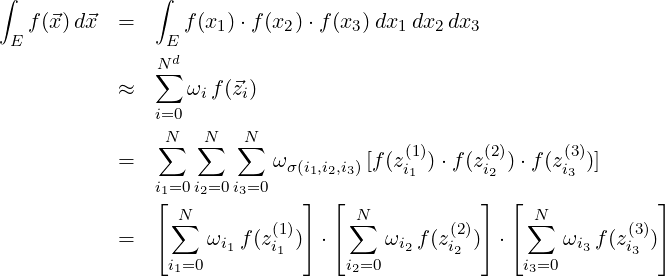As you can see, when the functions are separable and the quadrature (or collocating points) can be written in separable form, we can take O(Nd) operators and transform them into O(d ⋅ N)) operators. The above discussion is mainly focusing on the mathematical transformations needed to accomplish this; in subsequent sections, we will point out the memory layout and index ordering (i.e. now σ is ordered and implemented) to gain maximum performance.

The discussion of tensor-product operations on quadrilaterals and hexahedra may seem quite natural as the element construction is done with tensor-products of the segment; however, how do you create these types of operations on triangles (and anything involving triangles such as tetrahedra, prisms and pyramids)? The main mathematical building block we use that allows such operators is a transformation due to Duffy , which was subsequently used by Dubiner  in the context of finite elements and was extended to general polyhedral types by Ainsworth .

An image denoting the Duffy transformation is shown in Figure 5.3. On the left is an example of the right-sided standard triangle with coordinate system (ξ12), and on the right is the separable tensor-product domain with coordinates (η12). We often refer to this transformation as “collapsed coordinates" as it appears as the “collapsing" of a quadrilateral domain to a triangle. Note that the edge along η1 = 1 on the quadrilateral collapses down to the single point (ξ12) = (1,1). This, in general, does not cause issues with our integration over volumes as this is a point of measure zero. However, special case will need be taken when doing edge integrals. We will make it a point to highlight those places in which special care is needed.Figure 5.3 Duffy mapping between a right-sided triangle and a square domain.

With the 2D Duffy transformation in place, we can actually create all four 3D element types from the starting point of a hexahedra (our base tensor-product shape). One collapsing operation yields a prism. The second collapsing operation yields a pyramid. Lastly, a final collapsing operation yields a tetrahedra. A visual representation of these operations are shown in Figure 5.4.Figure 5.4 Duffy mapping application to generate all the 3D element types. Image taken from .

This is meant merely to be a summary of tensor-product operations, enough to allow us to discuss the basic data types and algorithms within Nektar++. If more details are needed, we encourage the reader to consult Karniadakis and Sherwin  and the references therein.

#### 5.1.2 Reference Elements On Primitive Geometric Types

Although the primary backbone of Nektar++ is tensor-product operations across all element types through the use of collapsed coordinates, we have implemented two commonly used non-tensorial basis set definitions. These are based upon Lagrange polynomial construction from a nodal (collocating) point set. As derived element types (in the C + + sense), we have implemented NodalStdTriangle (and Tet) based upon the electrostatic points of Hesthaven  and the Fekete points of Taylor and Wingate .

Although these types of elements, at first glance, might seem to be “sub-optimal" (in terms of operations), there are various reasons why people might choice to use them. For instance, the point set you use for defining the collocation points dictates the interpolative projection operator (and correspondingly the properties of that operator) – an important issue when evaluating boundary conditions, initial conditions and for some non-linear operator evaluations. Within the Nektar++ team, we started an examination of this within the paper by Kirby and Sherwin . There has since been various papers in the literature (beyond the scope of this developer’s guide) that give the pros and cons of tensor-product (separable) and non-tensor-product element types.